## Introduction

Studies of mobility patterns and predictions of individual mobility trajectories are important in many research fields, such as mobile computing, epidemic modeling, traffic planning and disaster response1,2,3. Real-time locations visited by individuals are typically collected through mobile devices equipped with global-positioning system (GPS) capability, mobile phone cell towers, or wireless local area network (WLAN) access points.

Various methods have been proposed to forecast individual trajectories, including Markov chain (MC) models4,5, neural networks6, Bayesian networks7 and finite automaton8. Prediction accuracy has been shown to vary according to the algorithm used and the context from which the location data come. For example, in an evaluation of next cell prediction based on more than 6000 users on Dartmouth's campus-wide Wi-Fi wireless network, it was found that the best predictor (the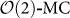model) had an accuracy of about 65–72%9. In another study where mobility traces of six researchers and GPS-locations of 175 individuals were used, the prediction accuracy was shown to be in the range of 70% to 95% with an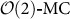model10,11,12. On the other hand, in an evaluation of MC models for pedestrian-movement prediction, the prediction accuracy was as low as 2%, 45% and 74.4% for the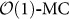model, hidden-Markov model and the mixed MC model, respectively13.

The above studies investigated small numbers of individuals or special populations and the results and practical feasibility of the proposed new predictive algorithms were therefore difficult to generalize to the general population. In addition, it was not clear how well these algorithms performed versus the best possible algorithm that could theoretically be constructed; i.e., what is, for the given data type, the best possible accuracy achievable and how well do the predictive algorithms perform versus such a benchmark? The highest potential accuracy of predictability, termed “maximum predictability” (Πmax), is defined by the entropy of information of a person's trajectory (frequency, sequence of location visits, etc.). Πmax can be calculated by solving a limiting case of Fano's inequality (a relation derived from calculation of the decrease in information in a noisy information channel)14,15,16.

By measuring Πmax, Song et al showed, using a mobile phone dataset of 50,000 users in a high-income country, that there is a 93% potential predictability in user mobility, despite very large differences in travel distances17. Under much more extreme conditions and in a low-income setting, Lu et al analyzed a complete mobile phone dataset of 2.9 million anonymous subscribers after the earthquake in Haiti in 2010 and found that despite massive population movements and increased travel distances following the earthquake, the predictability of people's movements remained as high as 85%, indicating a fundamental regularity in human mobility1. These findings are promising for the design and improvement of predictive algorithms. However, these studies did not show how close to the maximum potential predictability the accuracy of actual algorithms can come in practice.

In this study we aim to fill this gap in knowledge by measuring the maximum predictability and performance of actual prediction algorithms on a mobile phone data set of 500,000 users from Cote d'Ivoire (CIV), West Africa. We also give an overview of population mobility patterns during the data collection period, which took place after the 2011 civil war. We find that the maximum predictability and regularity in mobility in CIV is high, similar to what was found in studies in Haiti and Europe1,17. The evaluation of practical predictive algorithms on this dataset reveals that the maximum predictability can be approached with MC-based models. Interestingly, we also show that higher order MC models do not generate improved prediction accuracy when compared to a first order MC model.

## Results

### The mobile phone dataset

Mobility data was provided by the telecom company Orange and derived from call detail records (CDR) from a random sample of 500,000 anonymous Orange mobile phone subscribers, active during December 1, 2011 to April 28, 2012 in CIV. The user's location was provided as the location of the subprefecture (sous-préfecture in French) of the mobile phone tower through which the call was routed. CIV is composed of 19 regions, which are further divided into 255 subprefectures (237 of these subprefectures have at least one tower, see Fig. 1). The original CDR contains approximately five million users (1/4 of the total population of CIV)18,19. Detailed description of the data can be found in20.

The number of Orange subscriptions per person varies considerably throughout the country, as does the overall population density in CIV. For example, the region of Lagunes, which includes the economic capital Abidjan, is home to 25% of the CIV population and is the most frequently visited location for 43% percent of the mobile phone subscribers in this dataset (see Fig. 2A). The distribution of the number of location updates (calls and SMS) follows a log-normal distribution (Fig. 2B), with 81.3% having between 100 and 2000 location updates. Seventy-seven percent of users had at least one location update per day during two thirds of the data collection period (Fig. 2C). Heterogeneity in visitation patterns was high. While sixteen percent of subscribers were only found in one subprefecture during the period, a few users were registered in more than 50 subprefectures (Fig. 2D).

### Overview of mobility and aggregated flows

The absolute change in the number of subscribers in each region is dominated by the changes in the region of Lagunes, where Abidjan is situated (Fig. 3A). Seven-day cyclical patterns (workday-weekend cycles) are clearly visible for several regions, e.g. Lagunes and Sud-Comoe, but other more complex trends are also evident. An irregular change in population flow was observed near the end of March and early April when the numbers of users rapidly increased in Abidjan then decreased a few days later (potentially partly related to Easter). In addition, Bas-Sassandra, in the southwest experienced a decrease of users during large parts of the period studied here.

In relative terms (Fig. 3B), several regions showed considerable changes over the period of time analyzed, dominated by Denguele, which showed a small change in absolute terms (see Fig. 3A). As we see from Fig. 3C, both the distribution of average travel distances,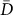and the radius of gyration, rg, (see Methods for definition) illustrate a skewed decay over increasing traveling distances. While the movements of the vast majority of users were confined within an area of 10 km, a few users traveled on average as far as 100 to 300 km per day (Fig. 3D). Note that the radius of gyration is calculated from location data on the level of the sub-prefecture and thus excludes short movements.

### Regularity and potential predictability

We now focus on the regularity of the daily observed trajectories of the users by allocating the last observed location (subprefecture) to each user's trajectory. To avoid the illusion of high predictability stemming from users with many unknown locations, or from users who never traveled to other locations, we include users who visited at least two subprefectures and were observable for more than 120 days in the period (208,288 users).

The distributions of users' random entropy (Srand), temporal-uncorrelated entropy (Sunc) and true-entropy (Sreal) are presented in Fig. 4A (see Methods). We can see that, consistent with findings from previous studies, the entropy of visited locations is greatly reduced if we consider both the spatial and temporal correlation of the visit sequences. The median value of Srand is 2.0, indicating that if we assume that individuals randomly choose a location to visit the next day, a typical individual could be found in any of 22.0 ≈ 4 locations. On the other hand, if we use information contained in the frequency and sequence order of the trajectory of individuals, the uncertainty in a typical individual's whereabouts reduces to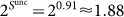and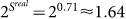, in less than two locations.

Not surprisingly, the reduced uncertainty leads to increased maximum predictability, as shown in Fig. 4B. If information is available only on the number of unique locations visited, Li, the accuracy of any predictive algorithm cannot exceed 0.35. With the additional information on frequency and temporal correlation, the average predictability increases to <Πunc> ≈ 0.84 and <Πmax> ≈ 0.88, respectively. Additionally, we evaluated the sensitivity of our entropy and predictability measures to the sampling rate of the data, without finding any important biases (see Supporting Information S1).

In Fig. 4C, we investigated the correlation between the radius of gyration and the average predictability, <Π>. There is a steady decrease of <Πrand> and <Πunc> when rg increases (measured based on the centroid of each subprefecture). On the other hand, <Πmax> stays around 0.85 for a wide range of rg [20, 300]. This finding is consistent with previous studies, revealing the independence of predictability on travel distance in human mobility1,17. However, we have also examined other travel distance measurements. Increases in average travel distance (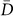) cause a slight decrease in predictability. <Πmax> ranging from 0.9 to 0.7 when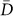increases from 1 to 20 km and stays around 0.63 0.68 for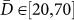. However, interestingly, predictability decreases considerably with an increasing number of unique locations visited. From Fig. 4E, we can see that the average predictability <Πunc> and <Πmax> decays almost linearly with the number of unique locations visited.

### Prediction accuracy based on Markov-chain models

The predictability analysis in the previous section reveals that, by combining information on frequency with temporal correlation of the trajectory, the theoretical upper bound of prediction accuracy can get as high as 0.88. However, the largest prediction accuracy that can be achieved with properly designed predictive algorithms is not given directly by this measure. In this section, we use MC(n) models to predict the location of users on each day, by considering all previous data points in the trajectory (see Methods). The accuracy of these models is presented in Fig. 5A and shows accuracies of more than 0.8 for almost all days. The accuracy of MC-based models (<γ> ≈ 0.91), MC(1) toMC(7), produce substantially higher accuracies than the estimation method based only on frequency information, i.e., MC(0) (<γ> ≈ 0.85).

There is however little difference between the performance of MC-based models of different orders. At the beginning of the period when historical information is limited, the accuracy of MC(1) is slightly higher than the other MC models, however this difference becomes very small when the historical trajectory is over 100 data points.

Another interesting finding from Fig. 5A, is that the MC-based models perform more robustly than MC(0). For example, during the later period of the data, there is a sharp decrease in the accuracy of MC(0) (from 0.88 to 0.77), while the accuracy of MC-based models shows a much smaller decrease, from 0.92 to 0.87. Irruptions of decreased accuracy from the MC(0) model indicate that people moved abnormally from their regular patterns. The sustainability of MC-based models reveals that such abnormalities can be captured partly by considering the temporal correlation of visiting sequences in the trajectories.

The increase of <γ> over the observation period is not very apparent from Fig. 5A, as <γ> is calculated based on a combination of users with long and short historical trajectories. To investigate the effect of trajectory length on the performance of algorithms, we removed, for each user, the unknown locations and calculated the average prediction accuracy for users with valid historical trajectories of the same length Lhist. The results (Fig. 5B) show that the accuracy of MC(0) approaches relative stability after around 15 historical data points. For a wide range of Lhsit [15, 120], <γ> is steady around 0.85, indicating that the visiting behavior on frequency is relatively stable over time for users with valid historical trajectories of this range. On the other hand, there is a steady increase of <γ> for the MC-based models. When the available historical trajectories contain more than 100 data points, the average prediction accuracy climbs to over 0.9.

The performance of MC-based models indicates that, while the predictability of a typical user is Πmax = 0.88, which gives an upper bound for the accuracy of any predictive algorithm when the trajectory is stable, the MC-based models are able to produce estimates as high as 0.91, even higher than the theoretical upper limit. Possible reasons for why the practical algorithm can produce accuracies higher than Πmax could be that the trajectory data contains only one data point for each day, which means that the maximum length of the trajectory can only be 150 and the movement patterns of individuals may not yet have stabilized. To investigate the effect of stability on the performance of prediction algorithms, we use the Geweke diagnostic21,22 to classify Xi into stationary and non-stationary trajectories (see Methods). In Fig. 6, we can see that there is a clear difference in the prediction accuracy of MC models between stationary and non-stationary trajectories: after 50 historical observations, the average prediction rate is about 0.95 for non-stationary trajectories and only 0.87 for stationary trajectories. This finding confirms that, given that the trajectory is stationary, the maximum predictability, Πmax, provides an upper bound of accuracy for any prediction algorithm. However, for non-stationary trajectories we show that prediction accuracy can greatly surpass the maximum predictability.

MC-models considering higher orders (longer correlations of previous locations) do not necessarily improve prediction accuracy. For example, for trajectories with the same historical length, the MC(4) model always produces less precise predictions compared to other MC-models (Fig. 5B). This finding is consistent with previous studies, in which the MC(n > 2) model was found to not bring important improvement at the cost of a significant overhead in terms of computation and space for the learning and storing of the mobility model9,12. It is worth noting that a large part of the predictive power of the studied prediction algorithm is due to the fact that many individuals spent a substantial time in his/her top visited locations. For example, users who visited four distinct subprefectures, still spent almost 80% of their time in their most visited locations (Fig. 5C).

### Entropy, predictability and prediction accuracy

The evaluation of predictive algorithms above reveal that, for this dataset, which is a combination of stationary and non-stationary trajectories, the maximum predictability Πmax can be achieved with a first-order Markov chain model (MC(1)). In this section, we investigate whether the individual predictability,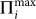, is correlated with the accuracy in predicting all the locations when the trajectory increases from 1 to T. We measure the individual prediction accuracy (<γi>) by the proportion of accurate predictions over all days for each individual (days without any location data are excluded).

First, we check the correlation between prediction accuracy and the disorder in the trajectory, i.e., Sreal. As we can see from Fig. 7A, <γi> is highly correlated with the trajectory's entropy; the larger the entropy, the lower the prediction accuracy. The correlation coefficient between Sreal and <γi> is as high as −0.849, with p < 0.000. Second, we investigate the correlation between prediction accuracy and the maximum predictability, i.e.,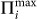. Not surprisingly,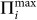also correlates highly with <γi>, with a correlation coefficient of 0.802, p < 0.000 (Fig. 7B).

The high correlation between predictability and prediction accuracy of the MC(1) model reveals that, as a measurement for disorder and potential predictability, Sreal and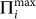capture the theoretical limits for the predictive analysis of human movement behaviors and provide an approachable upper bound of predictive power for this type of mobility data. More broadly, the approach used here provides an important strategy to evaluate and guide the design and improvement of mobility prediction algorithms.

## Discussion

By investigating the movement of 500,000 mobile phone users during the post-civil war period in Cote d'Ivoire, we have found a potential predictability in user mobility as high as 88% in this West-African, lower-middle income setting. The finding of high predictability is consistent with two previous studies which investigated the mobility patterns of mobile phone users in very different settings, one in a high-income country with stable social conditions17 and one in a low-income country following an extreme natural disaster1. By applying MC-based estimate algorithms, we found that the first order MC model (MC(1)) is able to produce an average predictive accuracy of 91%, with stationary and non-stationary trajectories having a predictive accuracy of 87% and 95% respectively.

One would perhaps assume that Markov chains of second or seventh order would produce next-location estimates with higher accuracy, as aggregated flows based on mobile phone data frequently show weekly cycles, see e.g., Fig. 3A, and1,2,17. However, our evaluations on the MC(n) models show that this information is not necessary in this setting. This could be due to the fact that many people in Cote d'Ivoire do not have a two-day weekend and that unplanned journeys are less common in resource-limited settings, which may be true of travel in general23,24. The trajectories used for prediction contain only the last observed location on each day, which makes it difficult for the time series to reach stability. As we can see, there is a big difference in the predictive power between stationary and non-stationary trajectories, implying that the diagnostics for convergence are critical for drawing conclusions from predictability analysis on human mobility. Nevertheless, we believe that the evaluation of predictive performance on a daily basis is most practical for the long-term investigation of population movements. For the purpose of this study, we have only included the Markov chain based models in the evaluation of predictive performance of algorithms. Future studies may want to compare other predictive algorithms, such as dynamic Bayesian networks (DBN)25, neural networks6 and finite automaton8 and to evaluate the feasibility of predicting aggregated population movements with individual-based travel behavior models.

In summary, this paper is, to the best of our knowledge, the first attempt to investigate both the maximum predictability and how close to this value practical algorithms can come when applied on a large mobile phone data set. Our results not only show that the predictability of human mobility is high, but also show that this high predictability is achievable for daily population movement predictions. These findings indicate that human mobility behavior is far from random and that individuals' movements are highly influenced by their historical behavior. With a good understanding of individuals' travel patterns, mobility modeling and public policy decision making, such as epidemic modeling, urban planning and traffic design, may be significantly improved.

## Methods

### Measures of mobility

We use the average travel distance,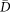and the radius of gyration of the trajectory, rg, to measure the mobility property of individuals. Specifically, let Mi = {m1, m2, …, mn} be the sequence of observed location updates for person i during the period of data collection. Then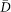and rg are defined by: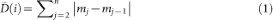and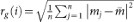, where |mjmj−1| is the distance between location mj and mj−1 and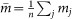is the center of mass of the trajectory26.

The radius of gyration is different from the average travel distance. Someone who moves in a comparatively confined space will have a small radius of gyration even though he or she covers a large distance. On the other hand, rg can be larger than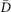if someone travels with small steps but in a fixed direction or in a large circle. Note that in the dataset used here we only know the location of each individual by subprefecture, consequently, the centroid of each subprefecture is used to approximate the coordinates of individuals. Such an approximation can introduce imprecision for the measure of travel distances, but still provides useful information when comparing mobility between users, as those who traveled across many subprefectures will have larger rg andthan those who spent most of their time in one or two subprefectures.

### Measures of entropy and predictability

We are primarily interested in the stable, long-term patterns of population mobility behavior as opposed to short-term movements. Here we focus on entropy and predictability analysis of day-to-day movements of individuals. Let Xi = {x1, x2, …, xT} be the sequence of daily locations for person i during the data collection period of T days. xj is the last observed location ID of person i on day j, otherwise we mark xj “unknown”. The uncertainty (or disorder) of the trajectories can be measured by entropy. Larger entropy indicates greater disorder and consequently reduces the predictability of an individual's movements.

#### Entropy

Following notation in17 we measure: (i) the random entropy,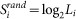, capturing the predictability of each user by assuming that the person's whereabouts are uniformly distributed among Li distinct locations in Xi; (ii) the temporal-uncorrelated entropy,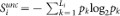, where pk is the frequency at which the person visited the kth location among the Li distinct locations.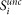takes into account the number of different locations visited as well as the proportion of time i spent at each location, decreasing the uncertainty of the trajectory, and; (iii) the true-entropy,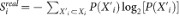, where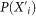is the probability of finding a sub-sequence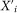in Xi, considering both spatial and temporal patterns.

#### Predictability

Given the entropy E for an individual i, Fano's inequality gives an upper limit for the predictability of i, i.e., the level of accuracy the best possible predictive algorithm can achieve: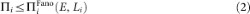where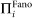is given by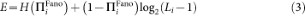and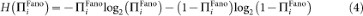Let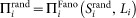,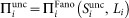and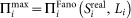, since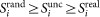, it is true that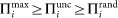. Comparing between these three predictability measurements provides us with the ability to investigate how the spatial distribution and temporal correlations in an individual's trajectory improve potential predictive power. Since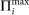provides the best possible predictive power (because it uses the maximum information from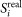) we refer to it in this paper as the “maximum predictability”.

### Prediction algorithms

#### Predicting a user's next location using Markov chain models

To investigate how close we can get to achieving Π with actual prediction algorithms we implement several variants of Markov chain (MC) based models.

In an MC-based model, the trajectory of each individual is modeled as a Markov chain of order n, which assumes that the movement of individuals between the Li locations is a process with limited memory in the sense that the future location is visited depending only on the previous n visited location, i.e.,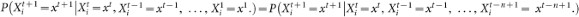, where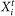is a random variable representing the location for individual i at time t.

Given the previous n locations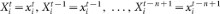, the prediction is then determined by the transition matrix, P, choosing the destination location xpre(1 ≤ preLi) which maximizes the probability: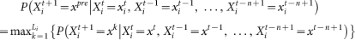Increases of the order n in the Markov chain do not necessarily lead to improvement in the prediction accuracy. However, to investigate the correlation of predictive powers with the length of trips to historical locations considered, we vary n from 1 to 7 (one day to one week). If predictions for a higher ordered MC(n) model did not exist (i.e., the order of the previous n locations is unique in history), the prediction from a lower ordered model, MC(n − 1), was used.

The performance of each model was evaluated by the accuracy, γ, which is the proportion of accurate predictions from all predictions made: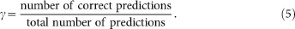Users who were not active on a specific day were excluded from the prediction.

#### Next place prediction using historical frequency data

For comparison we implemented a simple algorithm predicting the next location based on the most visited location in the historical trajectory: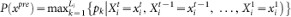, where pk is defined the same as in Sunc. As no temporal correlation is considered in this algorithm, we refer it as MC(0).

Using the MC models, we repeatedly updated the transition matrices and the visiting frequency for each user when new locations were observed in the trajectory. We predicted for each user the most likely location s/he would visit on each day based on all the historical information, i.e., for each day t, the transition matrices and visiting frequency are constructed based on the trajectory from day 1 to day t − 1.

### Geweke diagnostic

The Geweke Diagnostic21,22 is a test to detect failure of convergence by comparing values in the early part of a Markov chain to those in the latter part of the chain.

Let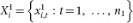and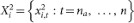, where 1 < n1 < na < n. let n2 = nna + 1 and define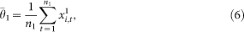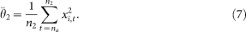Then the statistic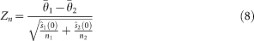converges to a standard normal distribution as n1 → ∞ given that the chain is stationary and (n1 + n2)/n < 1.

In the above equation,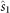and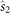denote consistent spectral density estimates at zero frequency21,22 for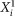and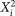, respectively.

Large Zn-scores then indicate rejection of the null hypothesis and provide evidence that the chain is non-stationary. For the purpose of this study, we first converted the values of xi,t into unique integers monotonically increasing from 1 and used a significant level of α = 0.05 and let n2/n = 50%. A trajectory is said to be stationary only if it passes all the tests at n1/n = 0.2, n1/n = 0.3, n1/n = 0.4 and n1/n = 0.5. By the end, the proportion of trajectories that passed the Geweke test (stationary trajectories) was 49%, see also supporting figure S2.